• 变量线性回归 import numpy as np import pandas as pd import matplotlib.pyplot as plt path = 'ex1data1.txt' data = pd.read_csv(path, header=None, names=['Population', 'Profit']) data.head()...
机器学习练习 1 - 线性回归
单变量线性回归
import numpy as np
import pandas as pd
import matplotlib.pyplot as plt

path =  'ex1data1.txt'


PopulationProfit06.110117.592015.52779.130228.518613.662037.003211.854045.85986.8233
data.describe()


PopulationProfitcount97.00000097.000000mean8.1598005.839135std3.8698845.510262min5.026900-2.68070025%5.7077001.98690050%6.5894004.56230075%8.5781007.046700max22.20300024.147000
看下数据长什么样子
data.plot(kind='scatter', x='Population', y='Profit', figsize=(12,8))
plt.show()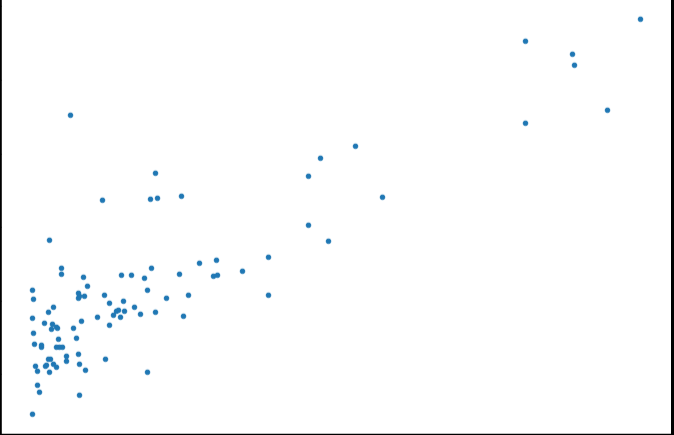现在让我们使用梯度下降来实现线性回归，以最小化成本函数。 以下代码示例中实现的方程在“练习”文件夹中的“ex1.pdf”中有详细说明。
首先，我们将创建一个以参数θ为特征函数的代价函数

J

(

θ

)

=

1

2

m

∑

i

=

1

m

(

h

θ

(

x

(

i

)

)

−

y

(

i

)

)

2

J\left( \theta \right)=\frac{1}{2m}\sum\limits_{i=1}^{m}{{{\left( {{h}_{\theta }}\left( {{x}^{(i)}} \right)-{{y}^{(i)}} \right)}^{2}}}

其中：${{h}{\theta }}\left( x \right)={{\theta }^{T}}X={{\theta }{0}}{{x}{0}}+{{\theta }{1}}{{x}{1}}+{{\theta }{2}}{{x}{2}}+…+{{\theta }{n}}{{x}_{n}}$
def computeCost(X, y, theta):
inner = np.power(((X * theta.T) - y), 2)
return np.sum(inner) / (2 * len(X))

让我们在训练集中添加一列，以便我们可以使用向量化的解决方案来计算代价和梯度。
data.insert(0, 'Ones', 1)

现在我们来做一些变量初始化。
# set X (training data) and y (target variable)
cols = data.shape
X = data.iloc[:,0:cols-1]#X是所有行，去掉最后一列
y = data.iloc[:,cols-1:cols]#X是所有行，最后一列

观察下 X (训练集) and y (目标变量)是否正确.
X.head()#head()是观察前5行


OnesPopulation016.1101115.5277218.5186317.0032415.8598
y.head()


Profit017.592019.1302213.6620311.854046.8233
代价函数是应该是numpy矩阵，所以我们需要转换X和Y，然后才能使用它们。 我们还需要初始化theta。
X = np.matrix(X.values)
y = np.matrix(y.values)
theta = np.matrix(np.array([0,0]))

theta 是一个(1,2)矩阵
theta

matrix([[0, 0]])

看下维度
X.shape, theta.shape, y.shape

((97, 2), (1, 2), (97, 1))

计算代价函数 (theta初始值为0).
computeCost(X, y, theta)

32.072733877455676


θ

j

:

=

θ

j

−

α

∂

∂

θ

j

J

(

θ

)

{{\theta }_{j}}:={{\theta }_{j}}-\alpha \frac{\partial }{\partial {{\theta }_{j}}}J\left( \theta \right)

def gradientDescent(X, y, theta, alpha, iters):
temp = np.matrix(np.zeros(theta.shape))
parameters = int(theta.ravel().shape)
cost = np.zeros(iters)

for i in range(iters):
error = (X * theta.T) - y

for j in range(parameters):
term = np.multiply(error, X[:,j])
temp[0,j] = theta[0,j] - ((alpha / len(X)) * np.sum(term))

theta = temp
cost[i] = computeCost(X, y, theta)

return theta, cost

初始化一些附加变量 - 学习速率α和要执行的迭代次数。
alpha = 0.01
iters = 1000

现在让我们运行梯度下降算法来将我们的参数θ适合于训练集。
g, cost = gradientDescent(X, y, theta, alpha, iters)
g

matrix([[-3.24140214,  1.1272942 ]])

最后，我们可以使用我们拟合的参数计算训练模型的代价函数（误差）。
computeCost(X, y, g)

4.5159555030789118

现在我们来绘制线性模型以及数据，直观地看出它的拟合。
x = np.linspace(data.Population.min(), data.Population.max(), 100)
f = g[0, 0] + (g[0, 1] * x)

fig, ax = plt.subplots(figsize=(12,8))
ax.plot(x, f, 'r', label='Prediction')
ax.scatter(data.Population, data.Profit, label='Traning Data')
ax.legend(loc=2)
ax.set_xlabel('Population')
ax.set_ylabel('Profit')
ax.set_title('Predicted Profit vs. Population Size')
plt.show()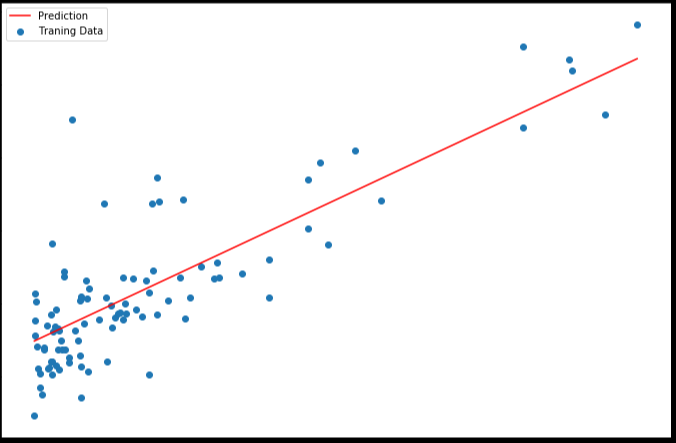由于梯度方程式函数也在每个训练迭代中输出一个代价的向量，所以我们也可以绘制。 请注意，代价总是降低 - 这是凸优化问题的一个例子。
fig, ax = plt.subplots(figsize=(12,8))
ax.plot(np.arange(iters), cost, 'r')
ax.set_xlabel('Iterations')
ax.set_ylabel('Cost')
ax.set_title('Error vs. Training Epoch')
plt.show()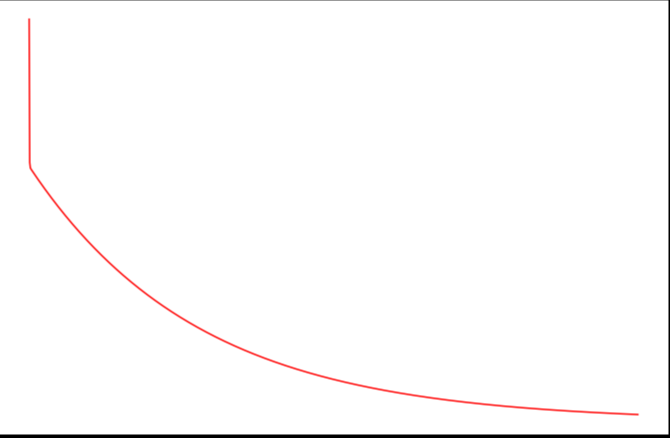多变量线性回归
练习1还包括一个房屋价格数据集，其中有2个变量（房子的大小，卧室的数量）和目标（房子的价格）。 我们使用我们已经应用的技术来分析数据集。
path =  'ex1data2.txt'


SizeBedroomsPrice021043399900116003329900224003369000314162232000430004539900
对于此任务，我们添加了另一个预处理步骤 - 特征归一化。 这个对于pandas来说很简单
data2 = (data2 - data2.mean()) / data2.std()


SizeBedroomsPrice00.130010-0.2236750.4757471-0.504190-0.223675-0.08407420.502476-0.2236750.2286263-0.735723-1.537767-0.86702541.2574761.0904171.595389
现在我们重复第1部分的预处理步骤，并对新数据集运行线性回归程序。
# add ones column
data2.insert(0, 'Ones', 1)

# set X (training data) and y (target variable)
cols = data2.shape
X2 = data2.iloc[:,0:cols-1]
y2 = data2.iloc[:,cols-1:cols]

# convert to matrices and initialize theta
X2 = np.matrix(X2.values)
y2 = np.matrix(y2.values)
theta2 = np.matrix(np.array([0,0,0]))

# perform linear regression on the data set
g2, cost2 = gradientDescent(X2, y2, theta2, alpha, iters)

# get the cost (error) of the model
computeCost(X2, y2, g2)

0.13070336960771892

我们也可以快速查看这一个的训练进程。
fig, ax = plt.subplots(figsize=(12,8))
ax.plot(np.arange(iters), cost2, 'r')
ax.set_xlabel('Iterations')
ax.set_ylabel('Cost')
ax.set_title('Error vs. Training Epoch')
plt.show()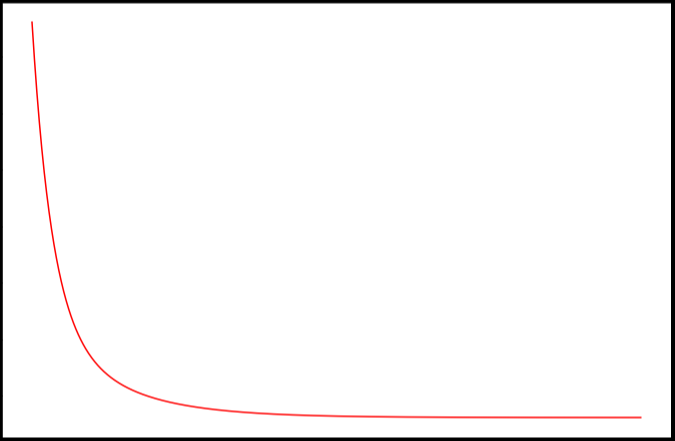我们也可以使用scikit-learn的线性回归函数，而不是从头开始实现这些算法。 我们将scikit-learn的线性回归算法应用于第1部分的数据，并看看它的表现。
from sklearn import linear_model
model = linear_model.LinearRegression()
model.fit(X, y)

LinearRegression(copy_X=True, fit_intercept=True, n_jobs=1, normalize=False)

scikit-learn model的预测表现
x = np.array(X[:, 1].A1)
f = model.predict(X).flatten()

fig, ax = plt.subplots(figsize=(12,8))
ax.plot(x, f, 'r', label='Prediction')
ax.scatter(data.Population, data.Profit, label='Traning Data')
ax.legend(loc=2)
ax.set_xlabel('Population')
ax.set_ylabel('Profit')
ax.set_title('Predicted Profit vs. Population Size')
plt.show()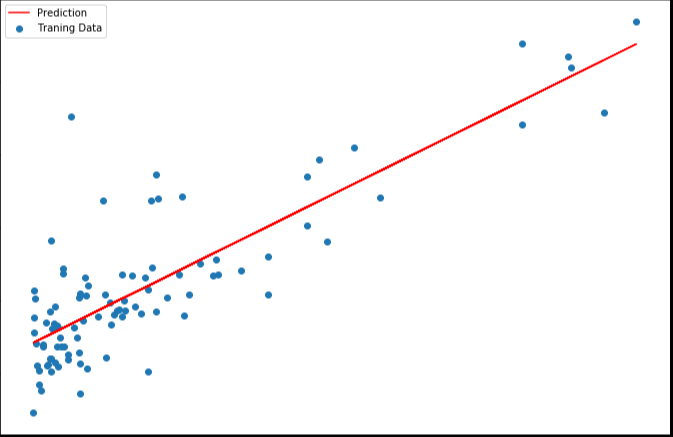4. normal equation（正规方程）
正规方程是通过求解下面的方程来找出使得代价函数最小的参数的：

∂

∂

θ

j

J

(

θ

j

)

=

0

\frac{\partial }{\partial {{\theta }_{j}}}J\left( {{\theta }_{j}} \right)=0

。 假设我们的训练集特征矩阵为 X（包含了

x

0

=

1

{{x}_{0}}=1

）并且我们的训练集结果为向量 y，则利用正规方程解出向量

θ

=

(

X

T

X

)

−

1

X

T

y

\theta ={{\left( {{X}^{T}}X \right)}^{-1}}{{X}^{T}}y

。 上标T代表矩阵转置，上标-1 代表矩阵的逆。设矩阵

A

=

X

T

X

A={{X}^{T}}X

，则：

(

X

T

X

)

−

1

=

A

−

1

{{\left( {{X}^{T}}X \right)}^{-1}}={{A}^{-1}}

梯度下降与正规方程的比较：
梯度下降：需要选择学习率α，需要多次迭代，当特征数量n大时也能较好适用，适用于各种类型的模型
正规方程：不需要选择学习率α，一次计算得出，需要计算

(

X

T

X

)

−

1

{{\left( {{X}^{T}}X \right)}^{-1}}

，如果特征数量n较大则运算代价大，因为矩阵逆的计算时间复杂度为

O

(

n

3

)

O(n3)

，通常来说当

n

n

小于10000 时还是可以接受的，只适用于线性模型，不适合逻辑回归模型等其他模型
# 正规方程
def normalEqn(X, y):
theta = np.linalg.inv(X.T@X)@X.T@y#X.T@X等价于X.T.dot(X)
return theta

final_theta2=normalEqn(X, y)#感觉和批量梯度下降的theta的值有点差距
final_theta2

matrix([[-3.89578088],
[ 1.19303364]])

#梯度下降得到的结果是matrix([[-3.24140214,  1.1272942 ]])

在练习2中，我们将看看分类问题的逻辑回归。


展开全文• matlab开发-不正则化多变量线性回归。线性回归演示
• ## 多变量线性回归

千次阅读 2016-12-04 11:03:40
目前为止,我们探讨了单变量/特征的回归模型,现在我们对房价模型增加更的特征, 例如房间数楼层等,构成一个含有变量的模型,模型中的特征为(x1,x2,…,xn)增添更特征后,我们引入一系列新的注释: n 代表特征的...
目前为止,我们探讨了单变量/特征的回归模型,现在我们对房价模型增加更多的特征, 例如房间数楼层等,构成一个含有多个变量的模型,模型中的特征为(x1,x2,…,xn)
增添更多特征后,我们引入一系列新的注释:  n 代表特征的数量

x(i)
$x^{(i)}$代表第 i 个训练实例,是特征矩阵中的第 i 行,是一个向量(vector)。

xij
$x^i_j$ 代表特征矩阵中第 i 行的第 j 个特征,也就是第 i 个训练实例的第 j 个特征。
支持多变量的假设 h 表示为:

h(x)=θ0+θ1x1+θ2x2...θnxn
$h(x)= \theta_0+\theta_1x_1+\theta_2x_2... \theta_nx_n$
这个公式中有 n+1 个参数和 n 个变量,为了使得公式能够简化一些,引入 x0=1,则公式  转化为:

h(x)=ΘTX
$h(x)=\Theta^TX$
多变量梯度下降
与单变量线性回归类似,在多变量线性回归中,我们也构建一个代价函数,则这个代价 函数是所有建模误差的平方和,即:

j(θ0,θ1...θn)=12m∑i=1m(hθ(x(i))−y(i))2

j(\theta_0,\theta_1...\theta_n)=\frac{1}{2m}\sum_{i=1}^{m}(h_\theta(x^{(i)})-y^{(i)})^2
其中:

hθ(x)=ΘTX=θ0x0+θ1x1+....θnxn
$h_\theta(x)=\Theta^TX=\theta_0x_0+\theta_1x_1+....\theta_nx_n$

我们的目标和单变量线性回归问题中一样,是要找出使得代价函数最小的一系列参数。  多变量线性回归的批量梯度下降算法为: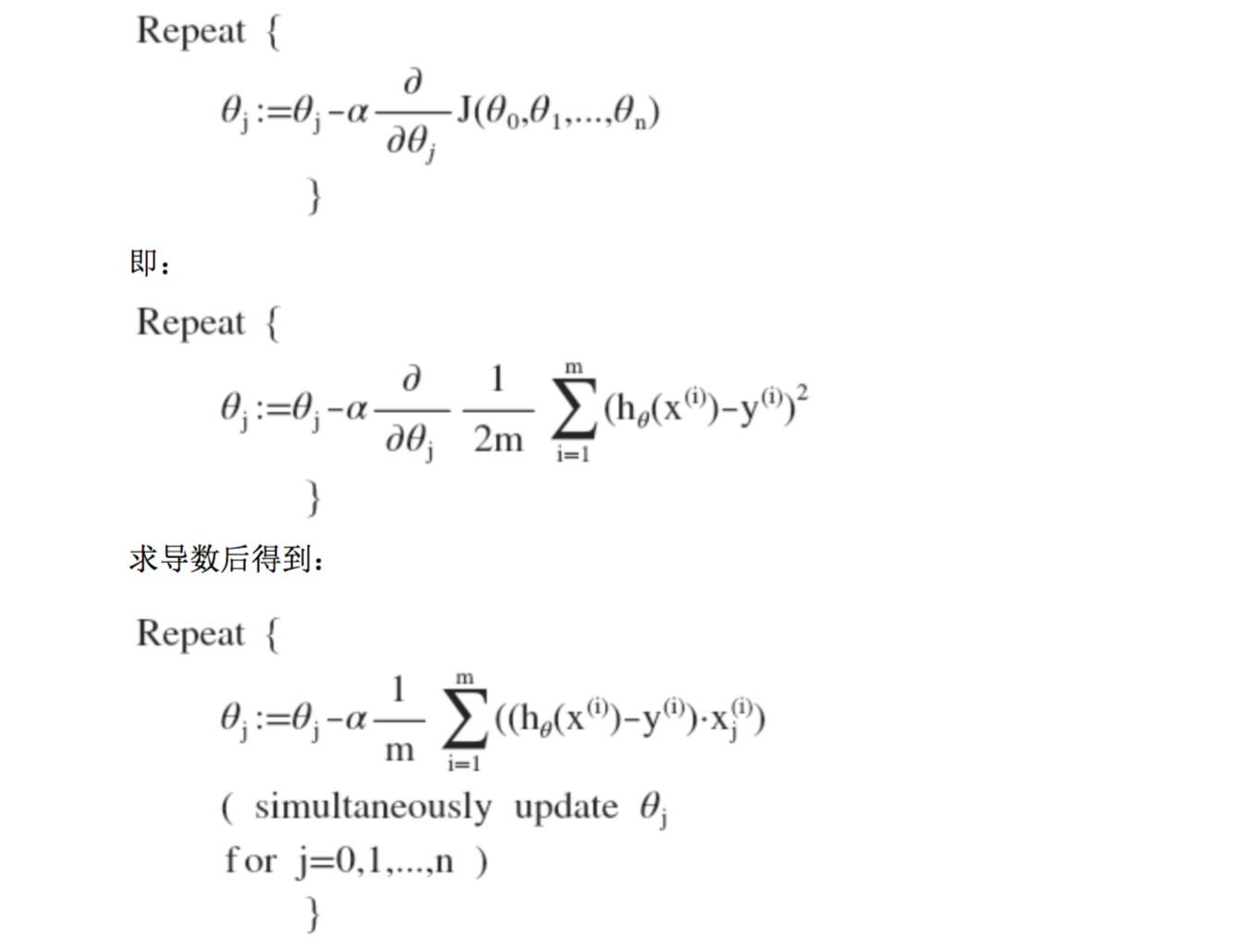梯度下降法实践 1-特征缩放
在我们面对多维特征问题的时候,我们要保证这些特征都具有相近的尺度,这将帮助梯 度下降算法更快地收敛。  以房价问题为例,假设我们使用两个特征,房屋的尺寸和房间的数量,尺寸的值为 0- 2000 平方英尺,而房间数量的值则是 0-5,以两个参数分别为横纵坐标,绘制代价函数的等 高线图能,看出图像会显得很扁,梯度下降算法需要非常多次的迭代才能收敛。
解决的方法是尝试将所有特征的尺度都尽量缩放到-1 到 1 之间。

xn=xn−unsn

x_n=\frac{x_n-u_n}{s_n}
其中

un
$u_n$表示平均值，

sn
$s_n$表示标准差

梯度下降法实践 2-学习率  梯度下降算法收敛所需要的迭代次数根据模型的不同而不同,我们不能提前预知,我们 可以绘制迭代次数和代价函数的图表来观测算法在何时趋于收敛。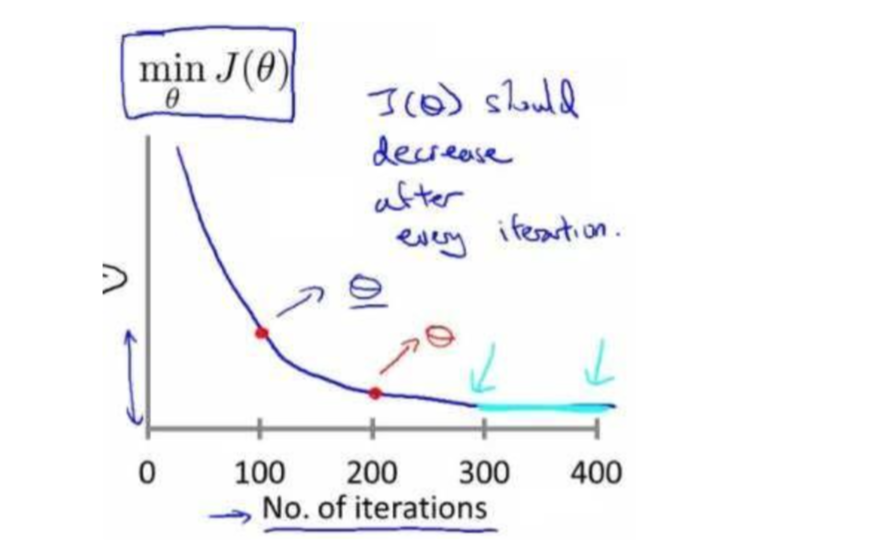也有一些自动测试是否收敛的方法,例如将代价函数的变化值与某个阀值(例如 0.001) 进行比较,但通常看上面这样的图表更好。  梯度下降算法的每次迭代受到学习率的影响,如果学习率 α 过小,则达到收敛所需的迭 代次数会非常高;如果学习率 α 过大,每次迭代可能不会减小代价函数,可能会越过局部最 小值导致无法收敛。
通常可以考虑尝试些学习率: α=0.01,0.03,0.1,0.3,1,3,10
特征和多项式回归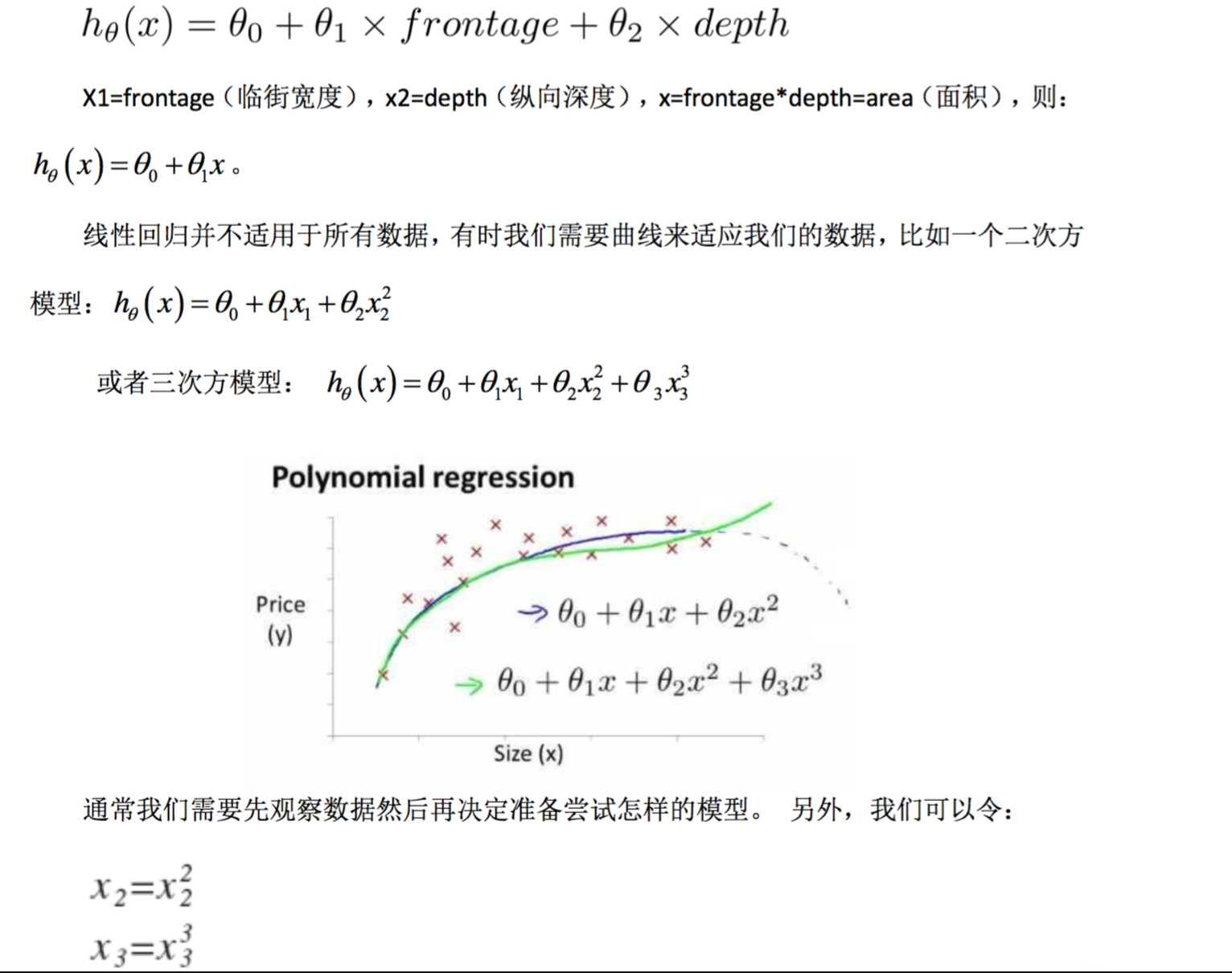注:如果我们采用多项式回归模型,在运行梯度下降算法前,特征缩放非常有必要。
正规方程
正规方程是通过求解下面的方程来找出使得代价函数最小的参数的:

σσθjJ(θj)=0
$\frac{\sigma}{\sigma\theta_j}J(\theta_j)=0$
假设我们的训练集特征矩阵为 X(包含了 x0=1)并且我们的训练集结果为向量 y,则利用正规方程解出的向量为

Θ=(XTX)−1XTy

\Theta=(X^TX)^{-1}X^Ty

注:对于那些不可逆的矩阵(通常是因为特征之间不独立,如同时包含英尺为单位的尺  寸和米为单位的尺寸两个特征,也有可能是特征数量大于训练集的数量),正规方程方法是 不能用的。
梯度下降与正规方程的比较: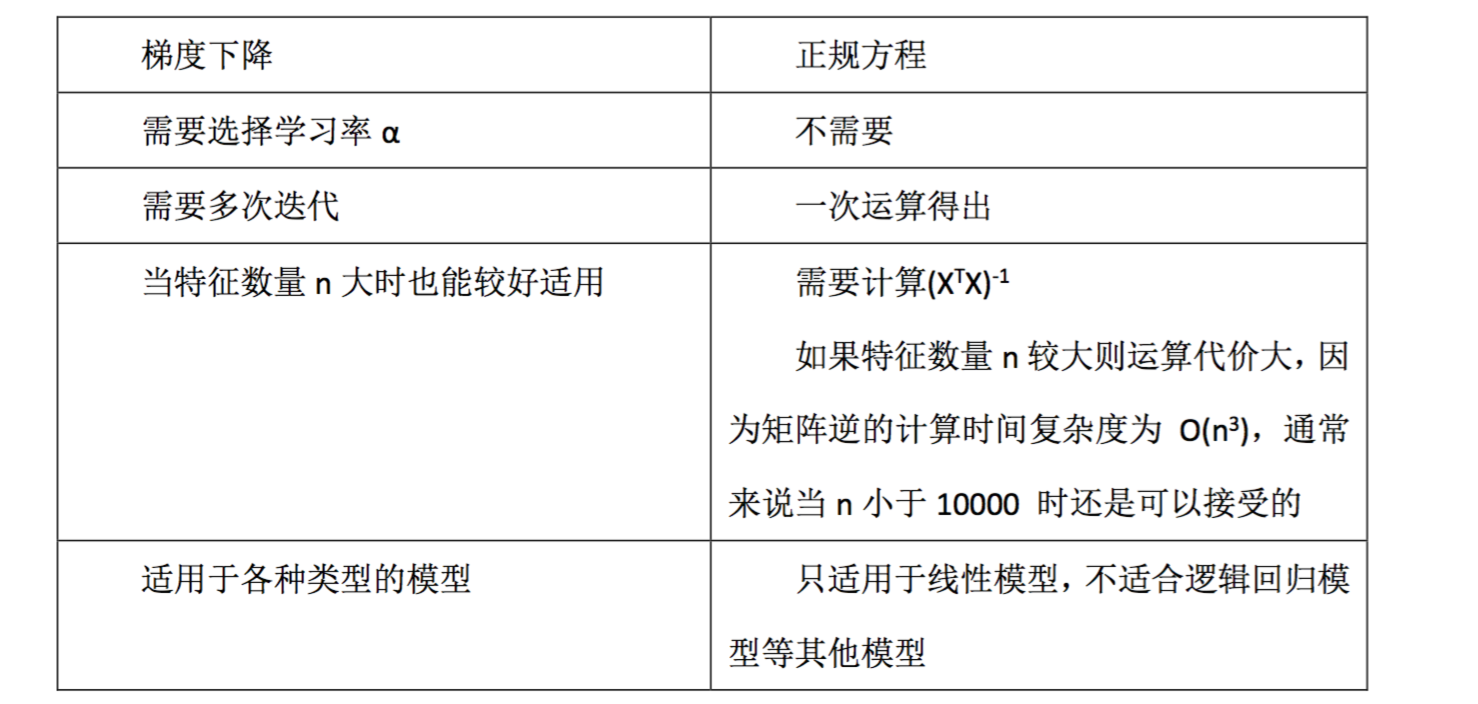总结一下,只要特征变量的数目并不大,标准方程是一个很好的计算参数 θ 的替代方 法。具体地说,只要特征变量数量小于一万,我通常使用标准方程法,而不使用梯度下降法。  随着我们要讲的学习算法越来越复杂,例如,当我们讲到分类算法,像逻辑回归算法,  我们会看到, 实际上对于那些算法,并不能使用标准方程法。对于那些更复杂的学习算法,  我们将不得不仍然使用梯度下降法。因此,梯度下降法是一个非常有用的算法,可以用在有大量特征变量的线性回归问题
正规方程及不可逆性
有些同学曾经问过我,当计算 θ=inv(X’X ) X’y ,那对于矩阵 X’X 的结果是不可逆的情况 咋办呢?  如果你懂一点线性代数的知识,你或许会知道,有些矩阵可逆,而有些矩阵不可逆。我 们称那些不可逆矩阵为奇异或退化矩阵。
问题的重点在于 X’X 的不可逆的问题很少发生,在 Octave 里,如果你用它来实现 θ 的 计算,你将会得到一个正常的解。在 Octave 里,有两个函数可以求解矩阵的逆,一个被称 为 pinv(),另一个是 inv(),这两者之间的差异是些许计算过程上的,一个是所谓的伪逆,另 一个被称为逆。使用 pinv() 函数可以展现数学上的过程,这将计算出 θ 的值,即便矩阵 X’X 是不可逆的。
在 pinv() 和 inv() 之间,又有哪些具体区别呢 ?  其中 inv() 引入了先进的数值计算的概念。例如,在预测住房价格时,如果 x1 是以英尺 为尺寸规格计算的房子,x2 是以平方米为尺寸规格计算的房子,同时,你也知道 1 米等于 3.28 英尺 ( 四舍五入到两位小数 ),这样,你的这两个特征值将始终满足约束:x1=x2* (3.28)2。
实际上,你可以用这样的一个线性方程,来展示那两个相关联的特征值,矩阵 X’X 将是 不可逆的。
第二个原因是,在你想用大量的特征值,尝试实践你的学习算法的时候,可能会导致矩 阵 X’X 的结果是不可逆的。  具体地说,在 m 小于或等于 n 的时候,例如,有 m 等于 10 个的训练样本也有 n 等于  100 的特征数量。
总之,出现不可逆矩阵的情况极少发生,所以 在大多数实现线性回归中,出现不可逆的问题不应该过多的关注 XTX 是不可逆的。
展开全文机器学习
•   问题 描述 表达式描述 表达式 单变量线性回归 只含有一个特征/输入... 多变量线性回归 含有个特征/输入变量 多元一次函数 多项式回归 含有个特征/输入变量 多元次函数 ...
https://blog.csdn.net/m511655654/article/details/81507857

问题描述表达式描述表达式单变量线性回归只含有一个特征/输入变量一元一次表达式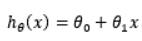多变量线性回归含有多个特征/输入变量多元一次函数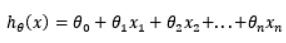多项式回归含有多个特征/输入变量多元多次函数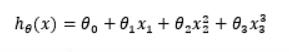逻辑回归含有多个离散输出，解决分类问题sigmoid函数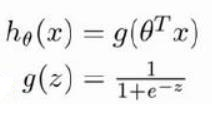过拟合有效适应训练集，但泛化性能差  1、剔除一些不必要特征，如PCA 2、正则化，保留所有特征，但减少参数的大小
问题梯度下降单变量线性回归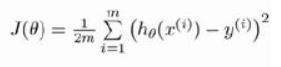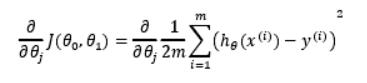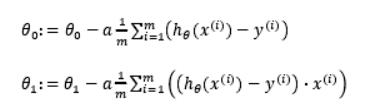多变量线性回归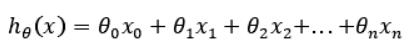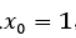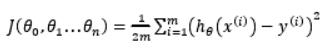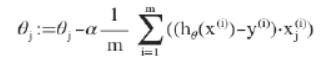特征缩放：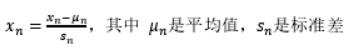学习率：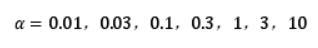逻辑回归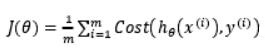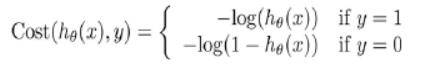等价于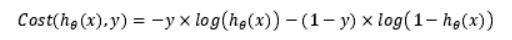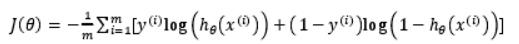求偏导得：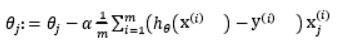正则化 修改代价函数：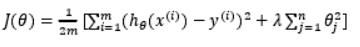令λ值很大，为了使cost function很小，所有θ（不包括θ0）都会一定程度较少。 正则化线性回归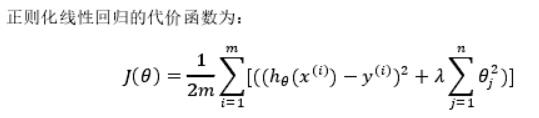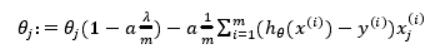正则化逻辑回归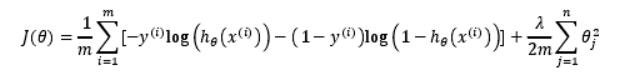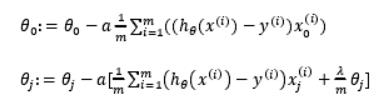展开全文机器学习 过拟合 正则化
• 机器学习(Machine Learning)是一门学科交叉专业，涵盖概率论知识，统计学知识以及复杂算法知识，使用计算机作为工具并致力于真实实时的模拟人类...一、单变量线性回归 提出问题 假设某披萨店的披萨价格和披萨...

机器学习(Machine Learning)是一门多学科交叉专业，涵盖概率论知识，统计学知识以及复杂算法知识，使用计算机作为工具并致力于真实实时的模拟人类学习方式， 并将现有内容进行知识结构划分来有效提高学习效率。本专栏将以学习笔记形式对《机器学习》的重点基础知识进行总结整理，欢迎大家一起学习交流！专栏链接：《机器学习》学习笔记

目录
一、单变量线性回归
提出问题
分析问题
解决方案
模型评价
二、多变量线性回归
1：基于LinearRegression的实现
2：基于成本函数和梯度下降的实现
三、数据评估之交叉验证法、留出法、自助法
1：SVM分类器
2：K近邻分类器

一、单变量线性回归
提出问题
假设某披萨店的披萨价格和披萨直径之间有下列数据关系：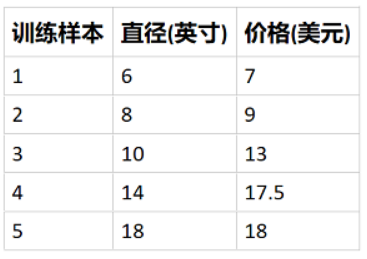根据上面的训练数据，我们能否推断(预测)出某个直径的披萨可能的售价呢？例如，12英寸的披萨可能售卖多少钱？
分析问题
把直径看成自变量𝑥(以后也称特征值)，价格看成因变量𝑦，可以先通过作图看出二者的关系：
import numpy as np
import matplotlib.pyplot as plt

def initPlot():
plt.figure()        #先准备好一块画布
plt.title('Pizza Price vs Diameter')  #表名字
plt.xlabel('Diameter')  #横坐标名字
plt.ylabel('Price')   #纵坐标名字
plt.axis([0, 25, 0, 25])        # 设置x轴和y轴的值域均为0~25
plt.grid(True)    #表内有栅格
return plt

plt = initPlot()    #画图
xTrain = np.array([6,8,10,14,18])
yTrain = np.array([7,9,13,17.5,18])
plt.plot(xTrain, yTrain, 'k.')  #k是黑色，.是以点作为图上显示
plt.show();    #将图显示出来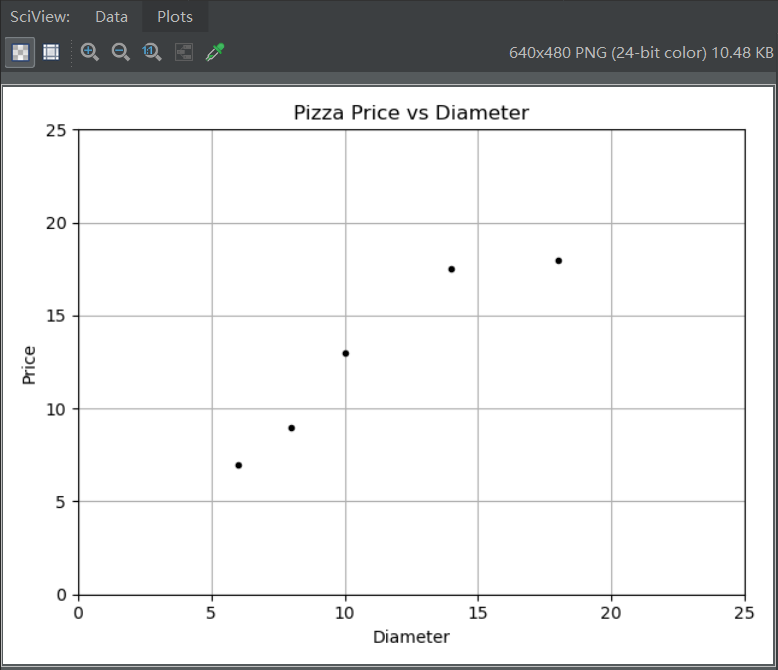可以看到：
价格𝑦随着直径𝑥的变化，大致呈现线性变化；如果根据现有的训练数据能够拟合出一条直线，使之与这些训练数据的各点都比较接近，那么根据该直线，就可以计算出在任意直径披萨的价格。
解决方案
采用Python scikit-learn库中提供的sklearn.linear_model.LinearRegression对象来进行线性拟合
思路 拟合出来的直线可以表示为：ℎ𝜃(𝑥)=𝜃0𝑥0+𝜃1𝑥1=𝜃0+𝜃1𝑥1hθ(x)=θ0x0+θ1x1=θ0+θ1x1
𝑥0x0 表示Intercept Term，一般设置为1即可𝑥1x1 表示影响计算结果的的第一个因素(或称特征，在本例中就是直径)。在单变量线性回归中，只有𝑥1x1𝜃0θ0表示截距，𝜃1θ1表示斜率。这两个参数都是需要通过拟合求出来的ℎ𝜃(𝑥)hθ(x)称为判别函数(Hypothesis Function)或判别式，也就是线性拟合的模型结果函数步骤
准备训练数据xTrain = np.array([6,8,10,14,18])[:, np.newaxis] yTrain = np.array([7,9,13,17.5,18]) LinearRegression支持单变量和多变量回归。对于多变量回归，xTrain显然是矩阵形式。因此，即使只有一个变量，LinearRegression也要求输入的特征值以矩阵形式(列向量)存在。 在使用LinearRegression时，不需要显式设置Intercept Item；它会自动扩展该列创建模型对象model = LinearRegression()执行拟合hypothesis = model.fit(xTrain, yTrain) 判别函数(hypothesis)对象中包含了大量的属性和方法，可用于针对该模型的后续操作获取判别函数的参数(截距和斜率)print("theta0=", hypothesis.intercept_) print("theta1=", hypothesis.coef_)预测新的数据model.predict([]) model.predict([,,,]) 将待预测的数据放置在一个矩阵(或列向量)中，可以批量预测多个数据结果 根据判别函数，绘制拟合直线，并同时显示训练数据点。 拟合的直线较好的穿过训练数据，根据新拟合的直线，可以方便的求出各个直径下对应的价格(预测结果)。
import numpy as np
import matplotlib.pyplot as plt
from sklearn.linear_model import LinearRegression

xTrain = np.array([6, 8, 10, 14, 18])[:, np.newaxis]  # 应以矩阵形式表达(对于单变量，矩阵就是列向量形式)
yTrain = np.array([7, 9, 13, 17.5, 18])  # 为方便理解，也转换成列向量

model = LinearRegression()  # 创建模型对象
hypothesis = model.fit(xTrain, yTrain)  # 根据训练数据拟合出直线(以得到假设函数)
print("theta0=", hypothesis.intercept_)  # 截距
print("theta1=", hypothesis.coef_)  # 斜率

print("预测直径12的披萨价格：", model.predict([]))  # 预测直径为12的披萨价格
xNew = np.array([0, 10, 14, 25])[:, np.newaxis]  # 也可以批量预测多个直径，注意要以列向量形式表达
yNew = model.predict(xNew)
print("预测新数据：", xNew)
print("预测结果：", yNew)

def initPlot():
plt.figure()
plt.title('Pizza Price vs Diameter')
plt.xlabel('Diameter')
plt.ylabel('Price')
plt.axis([0, 25, 0, 25])
plt.grid(True)
return plt

plt = initPlot()
plt.plot(xTrain, yTrain, 'k.')
plt.plot(xNew, yNew, 'g-')  # 画出通过这些点的连续直线
plt.show()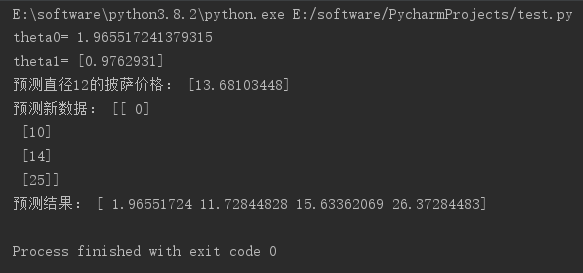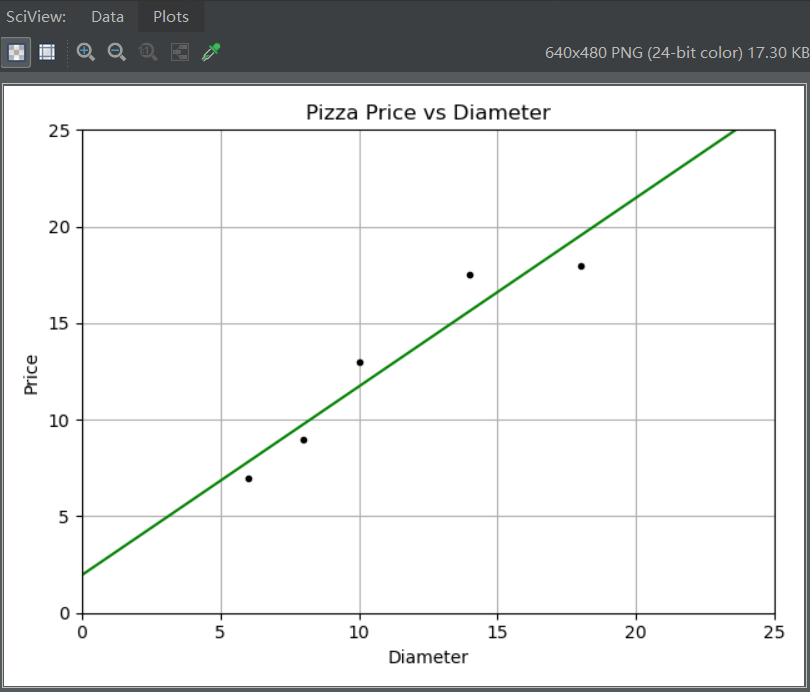模型评价
拟合出来的判别函数效果如何：对训练数据的贴合度如何？对新数据的预测准确度如何？ 先给出下列定义：
残差(residuals)：判别函数计算结果与实际结果之间的差异，如下图中的红色线段部分。一般是计算残差平方和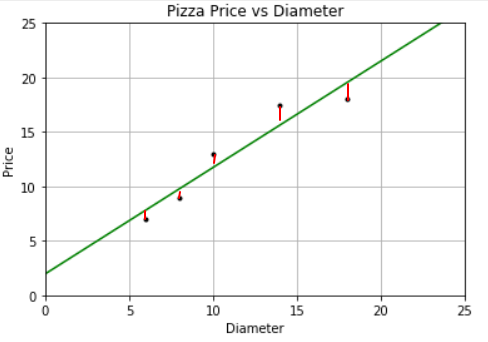R方(r-squared)：又称确定系数(coefficient of determination)。在通过训练数据得出了判别函数后，对于新的数据，如何评估该假设函数的表现呢？可以使用与训练数据不同的另一组数据（称为检验/测试数据）来进行评估。R方就是用来进行评估的一种计算方法。在Pyhton的scikit-learn中，是这样定义R方的（针对给定的测试数据）： 𝑆𝑆𝑡𝑜𝑡=∑𝑚𝑖=1(𝑦(𝑖)−𝑦⎯⎯⎯)2SStot=∑i=1m(y(i)−y¯)2 𝑆𝑆𝑟𝑒𝑠=∑𝑚𝑖=1[𝑦(𝑖)−ℎ𝜃(𝑥(𝑖))]2SSres=∑i=1m[y(i)−hθ(x(i))]2 𝑅2=1−𝑆𝑆𝑟𝑒𝑠𝑆𝑆𝑡𝑜𝑡R2=1−SSresSStot
𝑚m：测试数据集中的数据组数𝑦(𝑖)y(i)：测试数据集中第𝑖i组数据的𝑦y值（实际价格）𝑦⎯⎯⎯y¯：测试数据集中𝑦y的平均值ℎ𝜃(𝑥(𝑖))hθ(x(i))：将𝑥(𝑖)x(i)代入到判别函数计算的结果，也就是根据模型算出的𝑦y值（计算价格）𝑆𝑆𝑡𝑜𝑡SStot：针对测试数据计算出来偏差平方和𝑆𝑆𝑟𝑒𝑠SSres：针对测试数据计算出来的残差平方和一般来说，R方越大(不会超过1)，说明模型效果越好。如果R方较小或为负，说明效果很差在Python中如何对单变量线性回归模型的效果进行评估
手动计算 假设hpyTrain代表针对训练数据的预测𝑦y值，hpyTest代表针对测试数据的预测𝑦y值
训练数据残差平方和：ssResTrain = sum((hpyTrain - yTrain) ** 2)测试数据残差平方和：ssResTest = sum((hpyTest - yTest) ** 2)测试数据偏差平方和：ssTotTest = sum((yTest - np.mean(yTest)) ** 2)R方：Rsquare = 1 - ssResTest / ssTotTestLinearRegression对象提供的方法
训练数据残差平方和：model._residuesR方：model.score(xTest, yTest)
import numpy as np
import matplotlib.pyplot as plt
from sklearn.linear_model import LinearRegression

xTrain = np.array([6,8,10,14,18])[:,np.newaxis]         # 训练数据(直径)
yTrain = np.array([7,9,13,17.5,18])                     # 训练数据(价格)
xTest = np.array([8,9,11,16,12])[:,np.newaxis]          # 测试数据(直径)
yTest = np.array([11,8.5,15,18,11])                     # 测试数据(价格)

model = LinearRegression()
hypothesis = model.fit(xTrain, yTrain)
hpyTrain = model.predict(xTrain)
hpyTest = model.predict(xTest)                          # 针对测试数据进行预测

ssResTrain = sum((hpyTrain - yTrain)**2)                # 手动计算训练数据集残差
print(ssResTrain)                                       # 8.7478
print(model._residues)                                  # Python计算的训练数据集残差

ssResTest = sum((hpyTest - yTest)**2)                   # 手动计算测试数据集残差
ssTotTest = sum((yTest - np.mean(yTest))**2)            # 手动计算测试数据集y值偏差平方和
Rsquare = 1 - ssResTest / ssTotTest                     # 手动计算R方
print(Rsquare)                                          # 0.662
print(model.score(xTest, yTest))                        # Python计算的训练数据集的R方

# corrcoef函数是在各行元素之间计算相关性，所以x和y都应是行向量
print(np.corrcoef(xTrain.T, yTrain.T))            # 计算训练数据的相关性：0.954
print(np.corrcoef(xTest.T, yTest.T))              # 计算测试数据的相关性：0.816

def initPlot():
plt.figure()
plt.title('Pizza Price vs Diameter')
plt.xlabel('Diameter')
plt.ylabel('Price')
plt.axis([0, 25, 0, 25])
plt.grid(True)
return plt

plt = initPlot()
plt.plot(xTrain, yTrain, 'r.')          # 训练点数据(红色)
plt.plot(xTest, yTest, 'b.')            # 测试点数据(蓝色)
plt.plot(xTrain, hpyTrain, 'g-')        # 假设函数直线(绿色)
plt.show()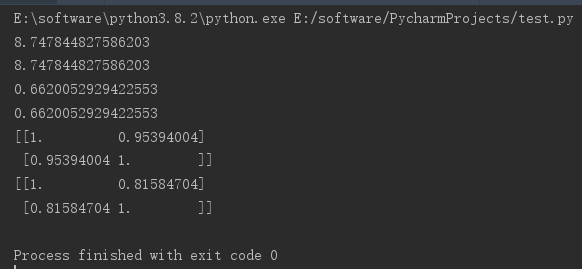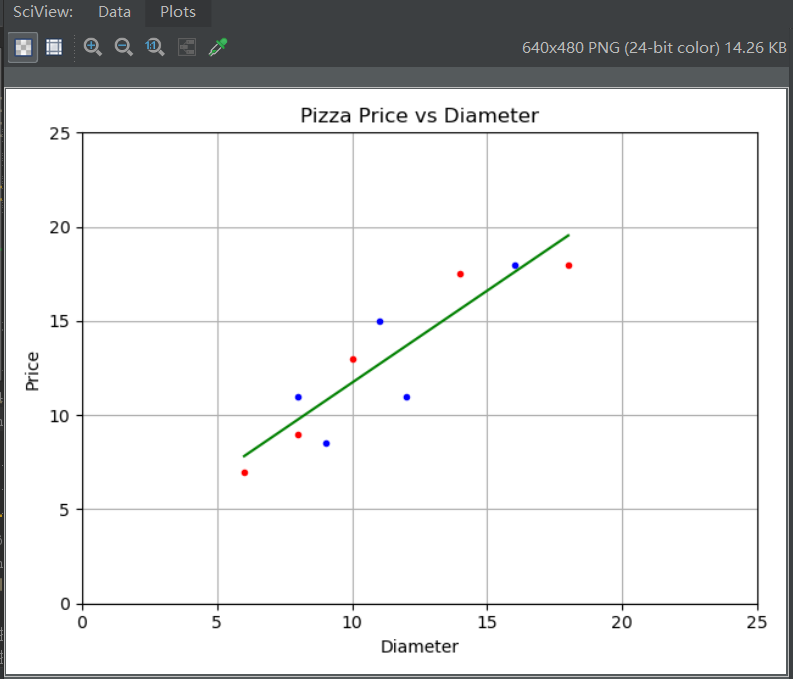二、多变量线性回归
在之前的但变量线性回归实验中，披萨价格仅与直径有关，按照这一假设，其预测的结果并不令人满意(R方=0.662)。本章再引入一个新的影响因素：披萨辅料级别(此处已经把辅料级别调整成数值，以便能够进行数值计算)。训练数据如下：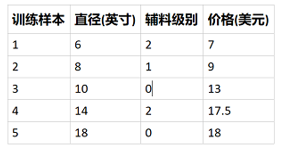另外提供测试数据如下：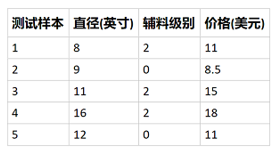如何使用线性回归训练数据，并且判断是否有助于提升预测效果呢？
1：基于LinearRegression的实现
与单变量线性回归类似，但要注意训练数据此时是(是训练数据条数，是自变量个数)，在本例中，是5x2的矩阵：xTrain = np.array([[6,2],[8,1],[10,0],[14,2],[18,0]])
针对测试数据的预测结果，其R方约为0.77，已经强于单变量线性回归的预测结果
''' 使用LinearRegression进行多元线性回归 '''

import numpy as np
from sklearn.linear_model import LinearRegression

xTrain = np.array([[6, 2], [8, 1], [10, 0], [14, 2], [18, 0]])  # 无需手动添加Intercept Item项
yTrain = np.array([7, 9, 13, 17.5, 18])

xTest= np.array([[8, 2], [9, 0], [11, 2], [16, 2], [12, 0]])
yTest = np.array([11, 8.5, 15, 18, 11])

model = LinearRegression()
model.fit(xTrain, yTrain)
hpyTest = model.predict(xTest)

print("假设函数参数：", model.intercept_, model.coef_)
print("测试数据预测结果与实际结果差异：", hpyTest - yTest)
print("测试数据R方：", model.score(xTest, yTest))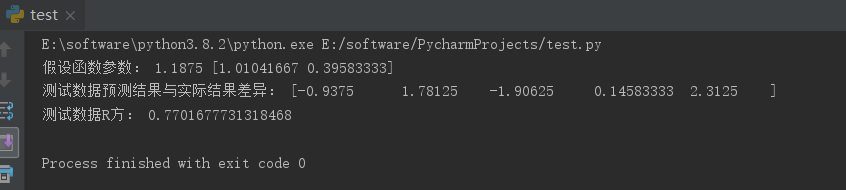2：基于成本函数和梯度下降的实现
对于一个自变量𝑥1的情形，𝑦与𝑥的关系用一条直线就可以拟合 (假设有一定线性相关性)。对于有两个自变量𝑥1,𝑥2x1,x2的情形， 𝑦与𝑥的关系就需要用一个平面来拟合。如果有更多的自变量，虽然 无法在三维空间中展现，但仍然可以用数学的方式来描述它们之间 的关系。
''' 批量梯度下降法实现多元线性回归 '''

import numpy as np
import matplotlib.pyplot as plt
import bgd_resolver

def costFn(theta, X, y):                           # 成本函数
temp = X.dot(theta) - y
return (temp.T.dot(temp)) / (2 * len(X))

def gradientFn(theta, X, y):                       # 根据成本函数，分别对x0,x1...xn求导数(梯度)
return (X.T).dot(X.dot(theta) - y) / len(X)

xTrainData = np.array([[6, 2], [8, 1], [10, 0], [14, 2], [18, 0]])
yTrain = np.array([7, 9, 13, 17.5, 18])
xTrain = np.c_[xTrainData, np.ones(len(xTrainData))]

np.random.seed(0)
init_theta = np.random.randn(xTrain.shape)
print("theta值", theta)

xTestData = np.array([[8, 2], [9, 0], [11, 2], [16, 2], [12, 0]])
yTest = np.array([11, 8.5, 15, 18, 11])
xTest = np.c_[xTestData, np.ones(len(xTestData))]
print("测试数据预测值与真实值的差异：", xTest.dot(theta) - yTest)

print("测试数据R方：", rsquare)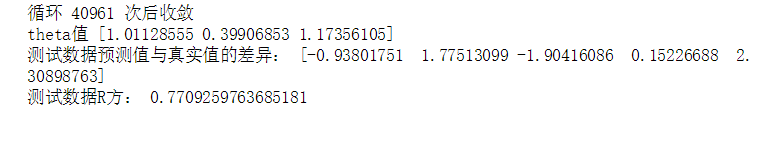三、数据评估之交叉验证法、留出法、自助法
1：SVM分类器
from sklearn.model_selection import train_test_split,cross_val_score,cross_validate # 交叉验证所需的函数(train_test_split对数据集和训练集做数据上的分割；cross_val_score做交叉验证；cross_validate也是做交叉验证)
from sklearn.model_selection import KFold,LeaveOneOut,LeavePOut,ShuffleSplit # 交叉验证所需的子集划分方法（KFold做k折交叉验证；LeaveOneOut留一法；LeavePOut留P个；ShuffleSplit打乱）
from sklearn.model_selection import StratifiedKFold,StratifiedShuffleSplit # 分层分割（StratifiedKFold使得分得的和原数据集中的比例及数目一致）
from sklearn.model_selection import GroupKFold,LeaveOneGroupOut,LeavePGroupsOut,GroupShuffleSplit # 分组分割
from sklearn.model_selection import TimeSeriesSplit # 时间序列分割
from sklearn import datasets  # 自带数据集
from sklearn import svm  # SVM算法（分类算法）
from sklearn import preprocessing  # 预处理模块
from sklearn.metrics import recall_score  # 模型度量（查全率、查准率）
import numpy as np

print('样本集大小：',iris.data.shape,iris.target.shape) #data是属性，targrt是标签，shape看大小

# ===================================数据集划分,训练模型==========================
X_train, X_test, y_train, y_test = train_test_split(iris.data, iris.target, test_size=0.4, random_state=0) #40%作为测试集 # 交叉验证划分训练集和测试集.test_size为测试集所占的比例
print('训练集大小：',X_train.shape,y_train.shape)  # 训练集样本大小
print('测试集大小：',X_test.shape,y_test.shape)  # 测试集样本大小
clf = svm.SVC(kernel='linear', C=1).fit(X_train, y_train) # 使用训练集训练模型
print('准确率：',clf.score(X_test, y_test))  # 计算测试集的度量值（准确率）

#  如果涉及到归一化，则在测试集上也要使用训练集模型提取的归一化函数。
scaler = preprocessing.StandardScaler().fit(X_train)  # 通过训练集获得归一化函数模型。（也就是先减几，再除以几的函数）。在训练集和测试集上都使用这个归一化函数
X_train_transformed = scaler.transform(X_train)
clf = svm.SVC(kernel='linear', C=1).fit(X_train_transformed, y_train) # 使用训练集训练模型
X_test_transformed = scaler.transform(X_test)
print('归一化后的准确率：', clf.score(X_test_transformed, y_test))  # 计算测试集的度量值（准确度）

# ===================================直接调用交叉验证评估模型==========================
clf = svm.SVC(kernel='linear', C=1)
scores = cross_val_score(clf, iris.data, iris.target, cv=5)  #cv为迭代次数。
print('交叉验证评估分数：', scores)  # 打印输出每次迭代的度量值（准确度）
print("Accuracy: %0.2f (+/- %0.2f)" % (scores.mean(), scores.std() * 2))  # 获取置信区间。（也就是均值和方差）

# ===================================多种度量结果======================================
scoring = ['precision_macro', 'recall_macro'] # precision_macro为精度（查准率），recall_macro为召回率（查全率）
scores = cross_validate(clf, iris.data, iris.target, scoring=scoring, cv=5, return_train_score=True)
sorted(scores.keys())  #排序
print('测试结果：',scores)  # scores类型为字典。包含训练得分，拟合次数， score-times （得分次数）

# ==================================K折交叉验证、留一交叉验证、留p交叉验证、随机排列交叉验证==========================================
# k折划分子集
kf = KFold(n_splits=2)
for train, test in kf.split(iris.data):
print("k折划分：%s %s" % (train.shape, test.shape))
break

# 留一划分子集
loo = LeaveOneOut()
for train, test in loo.split(iris.data):
print("留一划分：%s %s" % (train.shape, test.shape))
break

# 留p划分子集

lpo = LeavePOut(p=2)
for train, test in lpo.split(iris.data):
print("留p划分：%s %s" % (train.shape, test.shape))
break

# 随机排列划分子集
ss = ShuffleSplit(n_splits=3, test_size=0.25,random_state=0)
for train_index, test_index in ss.split(iris.data):
print("随机排列划分：%s %s" % (train.shape, test.shape))
break

# ==================================分层K折交叉验证、分层随机交叉验证==========================================
skf = StratifiedKFold(n_splits=3)  #各个类别的比例大致和完整数据集中相同
for train, test in skf.split(iris.data, iris.target):
print("分层K折划分：%s %s" % (train.shape, test.shape))
break

skf = StratifiedShuffleSplit(n_splits=3)  # 划分中每个类的比例和完整数据集中的相同
for train, test in skf.split(iris.data, iris.target):
print("分层随机划分：%s %s" % (train.shape, test.shape))
break

# ==================================组 k-fold交叉验证、留一组交叉验证、留 P 组交叉验证、Group Shuffle Split==========================================
X = [0.1, 0.2, 2.2, 2.4, 2.3, 4.55, 5.8, 8.8, 9, 10]
y = ["a", "b", "b", "b", "c", "c", "c", "d", "d", "d"]
groups = [1, 1, 1, 2, 2, 2, 3, 3, 3, 3]

# k折分组
gkf = GroupKFold(n_splits=3)  # 训练集和测试集属于不同的组
for train, test in gkf.split(X, y, groups=groups):
print("组 k-fold分割：%s %s" % (train, test))

# 留一分组
logo = LeaveOneGroupOut()
for train, test in logo.split(X, y, groups=groups):
print("留一组分割：%s %s" % (train, test))

# 留p分组
lpgo = LeavePGroupsOut(n_groups=2)
for train, test in lpgo.split(X, y, groups=groups):
print("留 P 组分割：%s %s" % (train, test))

# 随机分组
gss = GroupShuffleSplit(n_splits=4, test_size=0.5, random_state=0)
for train, test in gss.split(X, y, groups=groups):
print("随机分割：%s %s" % (train, test))

# ==================================时间序列分割==========================================
tscv = TimeSeriesSplit(n_splits=3)
TimeSeriesSplit(max_train_size=None, n_splits=3)
for train, test in tscv.split(iris.data):
print("时间序列分割：%s %s" % (train, test))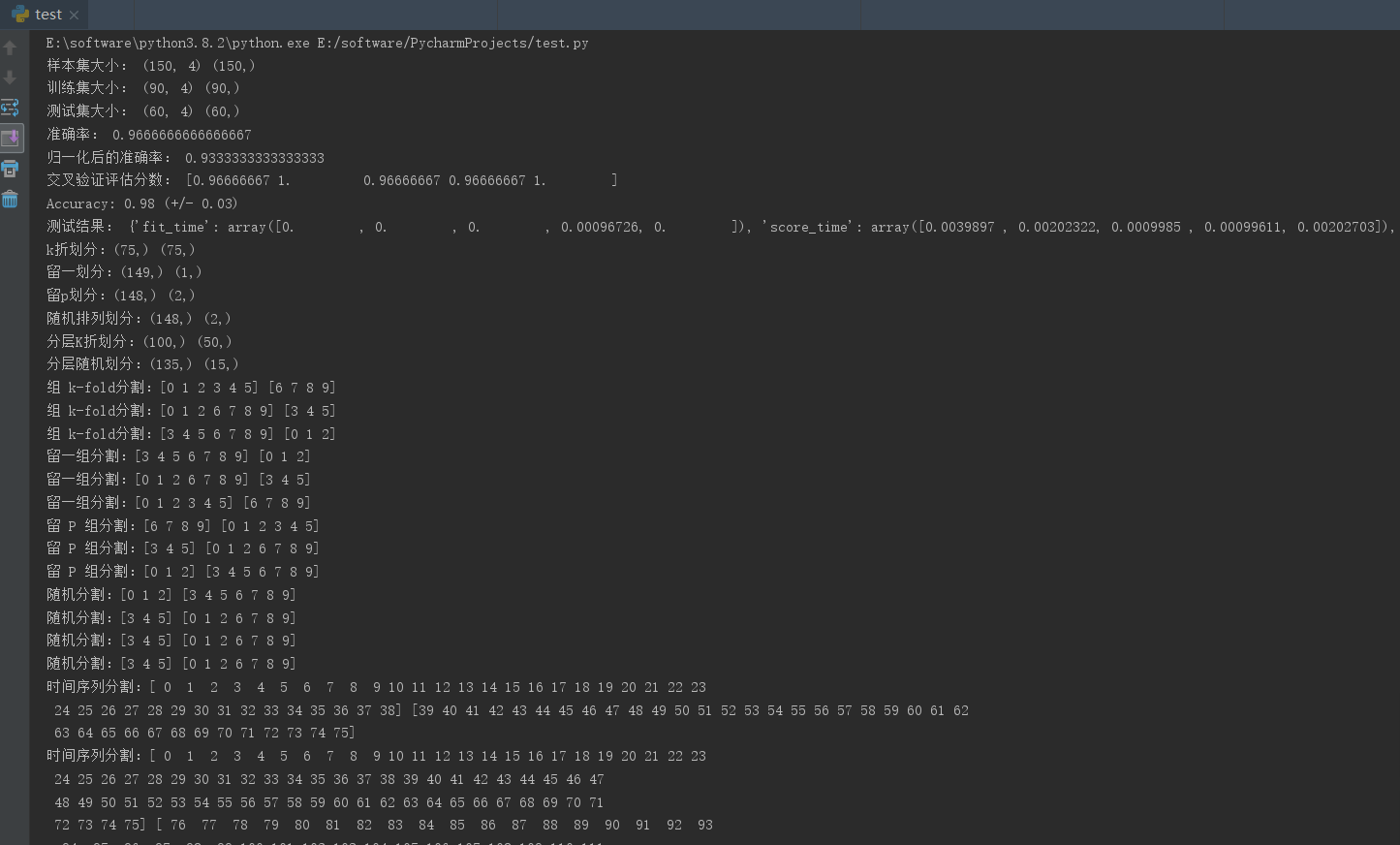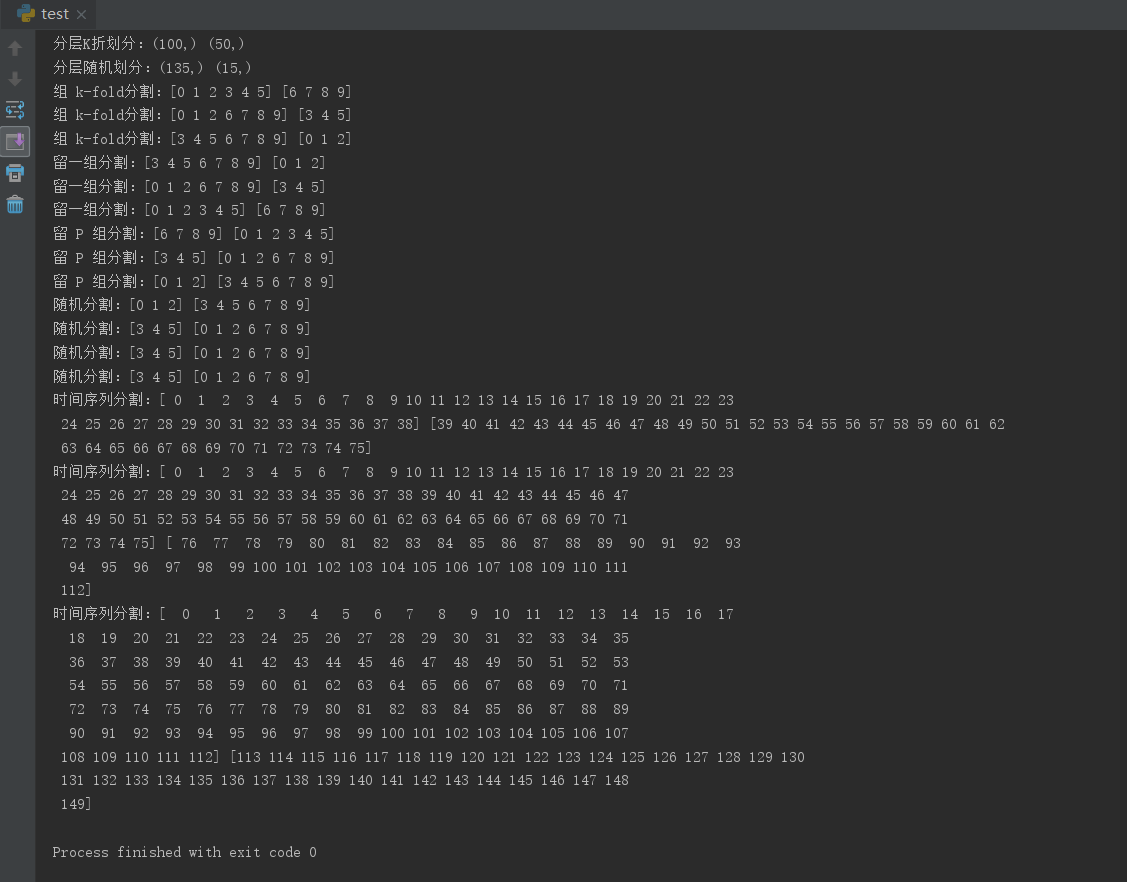2：K近邻分类器
from sklearn import datasets	#自带数据集
from sklearn.model_selection import train_test_split,cross_val_score	#划分数据 交叉验证
from sklearn.neighbors import KNeighborsClassifier  #一个简单的模型，只有K一个参数，类似K-means
import matplotlib.pyplot as plt

X = iris.data 			#这是数据
y = iris.target 		#这是每个数据所对应的标签

train_X,test_X,train_y,test_y = train_test_split(X,y,test_size=1/3,random_state=3)	#这里划分数据以1/3的来划分 训练集训练结果 测试集测试结果

k_range = range(1,31)
cv_scores = []		#用来放每个模型的结果值

for n in k_range:
knn = KNeighborsClassifier(n)   #knn模型，这里一个超参数可以做预测，当多个超参数时需要使用另一种方法GridSearchCV
scores = cross_val_score(knn,train_X,train_y,cv=10,scoring='accuracy') #accuracy准确率 #cv：选择每次测试折数  accuracy：评价指标是准确度,可以省略使用默认值，具体使用参考下面。
cv_scores.append(scores.mean())

plt.plot(k_range,cv_scores)
plt.xlabel('K')
plt.ylabel('Accuracy')		#通过图像选择最好的参数
plt.show()
best_knn = KNeighborsClassifier(n_neighbors=3)	# 选择最优的K=3传入模型
best_knn.fit(train_X,train_y)			#训练模型
print(best_knn.score(test_X,test_y))	#看看评分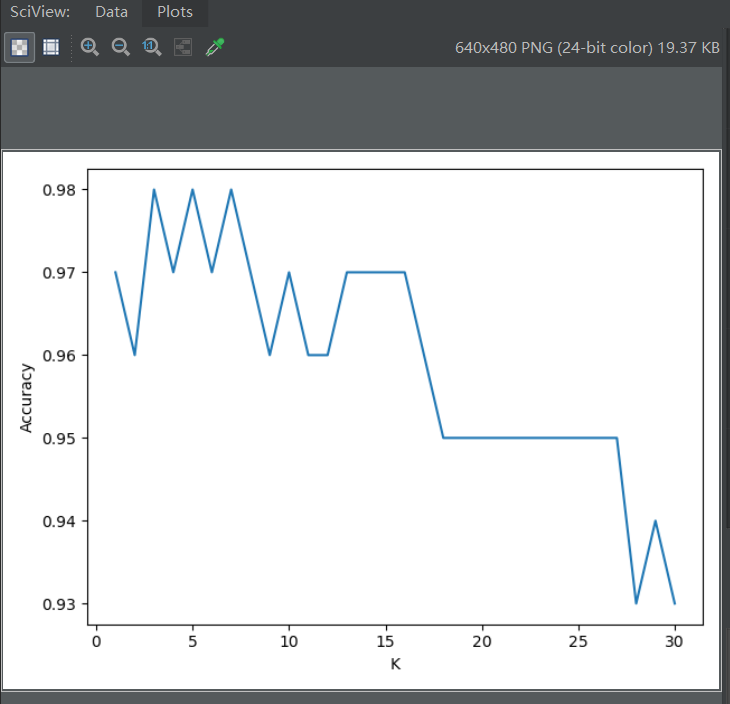欢迎留言，一起学习交流~~~
感谢阅读
END
展开全文python
• 文章目录第2章：单变量线性回归2.1 模型描述 Model representation2.2 代价函数 Cost function2.3 梯度下降 Gradient descent2.4 线性回归的梯度下降 Gradient descent for linear regression 第2章：单变量线性回归...
• ## 单变量线性回归

千次阅读 2016-04-08 12:40:51
变量线性回归就是从一个输入值预测一个输出值。输入/输出关系是一个线性函数。 模型表示： 我们用一个根据房屋尺寸预测房屋售价的例子开始。 假设有一个数据集，我们称作训练集，如下表所示 我们用来描述回归...数据挖掘 机器学习
• 文章目录单变量线性回归(Linear Regression with One Variable)2.1 模型表示2.2 代价函数2.3 代价函数的直观理解I2.4 代价函数的直观理解II 单变量线性回归(Linear Regression with One Variable) 2.1 模型表示 我们...算法 python 机器学习 人工智能 深度学习
• matlab开发-单变量线性回归梯度描述算法。一种实用的机器学习算法
• 本节将介绍一种更有效的线性回归形式。这种形式适用于多个变量个特征的情况。 目前为止，我们探讨了单变量/特征的回归模型，如下。用房屋面积x预测房子价格y。 下面的公式就是我们所说的“假设”，其中x就是...吴恩达 机器学习
• 四、多变量线性回归（Linear Regression with multiple variables） 4.1. 多维特征（Multiple features） 4.2. 变量梯度下降（Gradient descent for multiple variables） 4.3. 梯度下降法实践1——特征缩放...机器学习 吴恩达 机器学习笔记 人工智能
• 1.0.单变量线性回归 1）题目： 在本部分的练习中，您将使用一个变量实现线性回归，以预测食品卡车的利润。假设你是一家餐馆的首席执行官，正在考虑不同的城市开设一个新的分店。该连锁店已经在各个城市拥有卡车，...机器学习 吴恩达
• 摘要： 给出一个房价预测的例子，x轴是房子的大小，y轴是房子的价格，图中标注了一些房子作为数据集，而这些点被称为标注数据(labeled data)，利用这样的...Linear Regression with one Variable(单变量线性
• 其中Y为输出变量、响应变量、因变量、被解释变量；m为均值；e为不可控因子，可以理解为噪声。故模型等式右边是用X组成的函数去描述Y的均值，即模型是在平均的意义下去描述自变量与因变量间的关系...模型诊断 正态分布
• 关键词：线性回归、监督学习、模型、假设函数、代价函数、梯度递降、学习率、训练集一.前言前段时间在学习 Andrew Ng 的《机器学习课程》，个人认为这是一个非常适合新手学习机器学习的课程（即使你对线性代数，微...机器学习 梯度下降算法 监督学习
• 1、广义线性回归 广义线性模型有三个组成部分： (1) 随机部分, 即变量所属的指数族分布 族成员, 诸如正态分布, 二项分布, Poisson 分布等等. (2) 线性部分, 即 η = x⊤β. (3) 连接函数 g(µ) = η。 R 中的广义...
• 多元线性回归是研究一个连续型变量和其他个变量间线性关系的统计学分析方法，如果在自变量中存在分类变量，如果直接将分类变量和连续性变量统一纳入模型进行分析是有问题的，尤其是无序分类资料，即使进入了模型，...
• 本文主要介绍c++实现线性回归问题，实现房价的预测，参考链接：http://openclassroom.stanford.edu/MainFolder/DocumentPage.php?course=DeepLearning&doc=exercises/ex2/ex2.html   1、测试数据:房价的估计 2、...c++ 机器学习
• ## Python实现多元线性回归

万次阅读 多人点赞 2018-04-12 21:39:25
Python实现多元线性回归 ...回归分析中，只包括一个自变量和一个因变量，且二者的关系可用一条直线近似表示，这种回归分析称为一元线性回归分析。如果回归分析中包括两个或两个以上的自变量，且因变...Python 数据分析 数据挖掘 pandas
• 斯坦福大学机器学习第二课"单变量线性回归“学习笔记，本次课程主要包括7部分： 1) Model representation(模型表示) 2) Cost function(代价函数，成本函数) 3) Cost function intuition I(直观解释1) 4) Cost ...
• 1、回归：预测一个具体的数值输出 2、分类：预测离散值输出 先来看机器学习是如何进行的： 其中，h表示假设函数： θ是参数，下一节我们谈谈如何选择这两个参数值。 二、代价函数（Cost function） 我们...
• 1. 假设函数之前的几篇文章里面，我们都只是介绍了单维特征变量线性回归模型，比如预测房价的时候，我们只用了房子的面积这个维度。...如下图因此，个维度特征变量线性回归的假设函数可定义为还是假
•  x：输入变量，也就是特征，可以有个，对于单变量线性回归只有一个输入。  y：输出变量，即目标值。  (x, y)：训练样本集。  (x (i) , y (i) )：第i个训练样本。  线性回归的目标是根据给定的...算法 system 任务
• 机器学习入门系列二（关键词：多变量(非)线性回归，批处理，特征缩放，正规方程） 目录(?)[+] 一多变量线性回归二批处理三特征缩放四正规方程五多变量线性回归 一、多变量线性...
• 今年在用机器学习分类的时候，发现很机器学习基础知识都已经忘得一干二净，对自己很是无语。因此，作者打算重新温习一篇Andrew老师的机器学习课程，并用博客来记录每一节课程的知识要点，加油吧！   机器学习...
• 该文章是吴恩达《机器学习》课程第一章的笔记。通过一元变量线性回归来讲解梯度下降算法的基础。机器学习
• 当存在分类变量时，需要首先处理成个哑变量，例如将年龄分成三个:age<18, age18_60, age>60。 然后在SPSS里纳入除了ref的所有哑变量，例如我们要将18-60作为参考组，就纳入age<18和age>60，不要纳入......